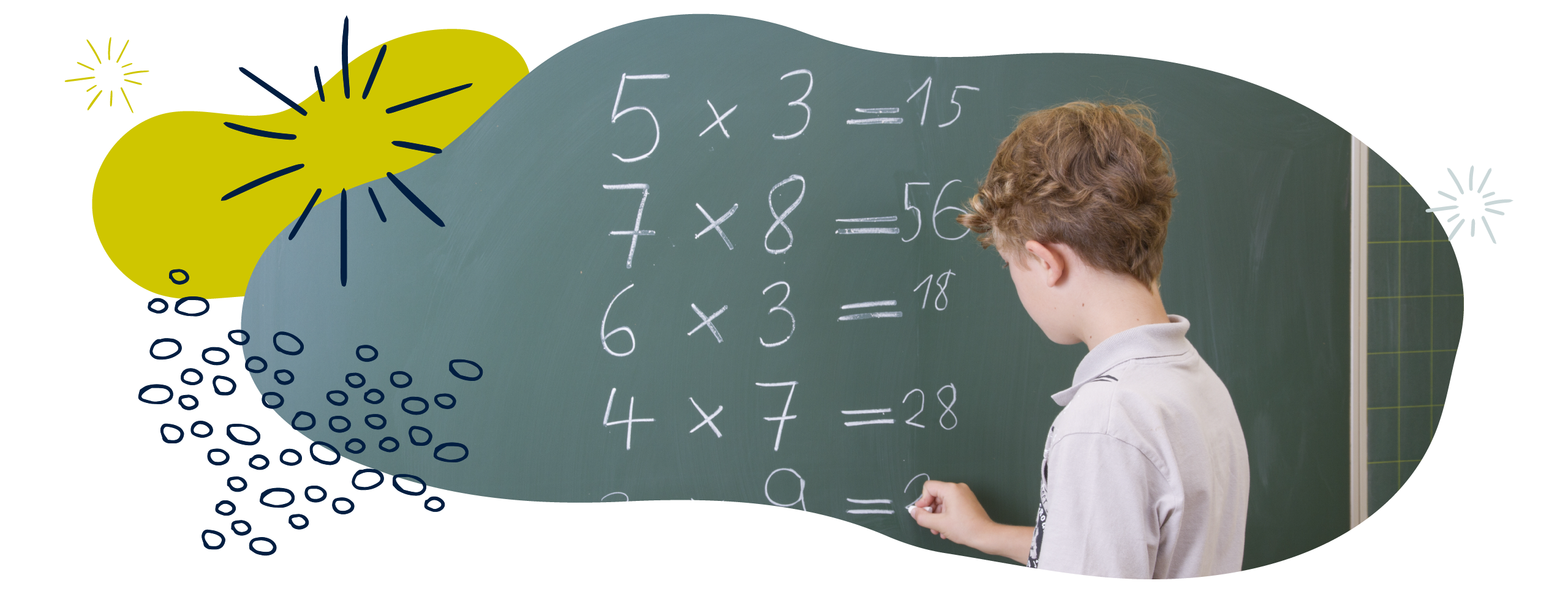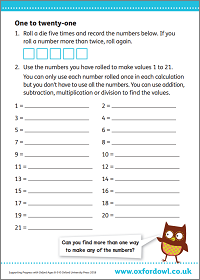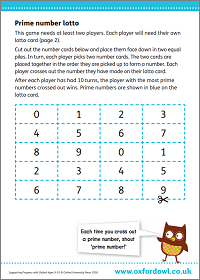We use cookies to enhance your experience on our website. By continuing to use our website, you are agreeing to our use of cookies. You can change your cookie settings at any time. Find out more# Multiplication & division in Year 5 (age 9–10)

In Year 5, your child will learn to identify multiples and factors, including finding all factor pairs of a number, common factors of 2 numbers, and prime numbers. They will solve increasingly complex problems.

The key words for this section are common factor, factor, and factor pair.

## What your child will learn

Take a look at the National Curriculum expectations for multiplication and division in Year 5 (ages 9–10):

#### Identify multiples and factors (including factor pairs and common factors)

Your child will understand and use the terms factor and factor pair. They will be introduced to common factors and should be able to use them in their calculations.

Your child will need to be able to recognise multiples and factors. For example, the factors of 12 are 1, 2, 3, 4, 6, and 12. The multiples of 12 are 12, 24, 36, and so on.

They should also be able to find factor pairs. Factor pairs are pairs of numbers that multiply together to make a third particular number. For example, the factor pairs of 12 are 1 and 12, 2 and 6, and 3 and 4.

They should also be able to find common factors of two numbers. For example, the factors of 12 are 1, 2, 3, 4, 6, and 12. The factors of 10 are 1, 2, 5, and 10. The common factors are the factors that are common to both numbers. So, the common factors of 10 and 12 are 1 and 2.

#### Use the vocabulary of prime numbers, prime factors, and composite numbers

Your child will be expected to use and understand the terms:

Factor – a whole number that can be multiplied by another whole number to make a particular third whole number. For example, 1, 2, 3, 6, 9, and 18 are all factors of 18.

Multiple – a whole number that can be divided by another whole number a certain number of times without a remainder. For example, multiples of 12 are 12, 24, 36, 48, 60, and so on.

Prime number – a number that only has factors of 1 and itself. A prime number can only be divided by 1 or itself to get a whole number. 2, 3, 5, 7, 11, and 13 are all prime numbers.

Composite number – a number with more than two factors. 4, 6, 8, 9, 10, and 12 are all composite numbers.

Square number – a number created by multiplying a whole number by itself. For example, 9 is a square number because it is the product of 3 × 3.

Cube number – a number created by multiplying a whole number by itself, and then by itself again. For example, 27 is a cube number because it is the product of 3 × 3 × 3.

#### Know the prime numbers up to 19 and work out if a number is prime up to 100

Your child will be expected to know all the prime numbers up to 19 (2, 3, 5, 7, 11, 13, 17, and 19). These are all numbers that only have two factors: itself and 1.

Your child should be able to work out whether any number up to 100 is a prime number using their knowledge of multiplication and factors.

#### Multiply and divide numbers mentally

Your child should know times tables and related division facts by heart and use them in larger calculations. They will use a variety of mental calculation methods and will be able to explain how to solve problems.

#### Multiply four-digit numbers by one- and two-digit numbers

Your child will continue to practise formal written methods of multiplication. They will use short multiplication to solve calculations involving four-digit numbers being multiplied by one-digit numbers (for example, 1368 × 6).

They will also learn the formal written method of long multiplication for calculations involving two-digit numbers (for example, 68 × 26).

#### Divide four-digit numbers by one-digit numbers

Your child will practise a greater variety of methods to solve division problems. These should include sharing and grouping using objects, chunking, and formal written methods of division.

They will divide numbers up to four digits by a one-digit number. For example, 2345 ÷ 5 = 469.

Your child will need to solve problems where the answer is not a whole number. They will be expected to use remainders appropriately, depending on the problem. For example:

If there are 19 children, and 5 children can fit in a car, how many cars are needed? The answer would be 4, even though the exact answer to 19 ÷ 5 is 3.8. Children should know that the question requires them to round the answer to 4, as you can’t travel in 0.8 of a car.

#### Multiply and divide numbers by 10, 100, and 1000

Your child will be expected to use their knowledge of place value to multiply and divide whole and decimal numbers by 10, 100 and 1000. For example, 395.5 ÷ 10 = 39.55.

#### Recognise and use square numbers and cube numbers

Your child will use square and cube numbers when calculating, especially when learning about area and volume. They will be able to use the correct notation (for example, 5² for five squared and 5³ for five cubed).

#### Use factors, multiples, squares, and cubes to solve problems

Your child will be expected to solve problems involving multiplication and division including using their knowledge of factors, multiples, squares, and cubes.

#### Solve problems with combinations of addition, subtraction, multiplication, and division

Your child will be able to use a variety of methods to solve multiplication and division problems and explain how they have solved problems. They will solve problems that include combinations of different operations.

Your child will be expected to use the equals sign (=) to indicate equivalence, including in missing number problems (for example, 13 + 24 = 12 + 25, and 33 = 5 × ?).

#### Solve problems by scaling simple fractions and using simple rates

Your child will be familiar with thinking about multiplication as scaling. Scaling is used when we use multiplication to change the size of the original quantity. For example:

‘If you are 5 years old and I am 6 times older than you, how old am I?

They will apply this knowledge to solving problems involving scaling by simple fractions. For example, if a product gets a 50% reduction in price.

Your child will also be expected to solve problems involving rate. Rate is a ratio that compares quantities measured in different units. For example, if a 200g can of baked beans costs 65p, the rate is 65p for 200g. They could be asked questions such as:

If it took 7 hours to mow 4 football pitches, then how many pitches could be mowed in 35 hours?

20 pitches

At what rate were pitches being mowed?

⅔ pitch per hour, or 1 pitch every 40 minutes

## How to help at home

There are lots of quick and easy ways that you can help your child to understand multiplication and division. Here are just a few ideas to support your child’s learning:

### 1. Multiplication game with dice

Make ‘×’, ‘÷’, ‘10’, ‘100’, and ‘1000’ cards. Place them face down in front of you in two piles.

Player A rolls a dice four times to create a four-digit number (for example, 4258). Player B must choose where to insert a decimal point to make a decimal number. Player A chooses a multiply or divide card at random and one of the number cards at random. They multiply or divide to make a new number. Then player B has a turn.

The player who makes the largest number wins the point. Play until one player reaches ten points.

### 2. Play Operation Challenge game

You will need to create two sets of number cards: 1–10 (the small numbers), and the numbers 25, 50, 75, and 100 (the large numbers).

1. Arrange the cards face down into two groups: ‘Large numbers’ and ‘small numbers’.
2. Ask your child to make up a random three-digit target, like 274 or 833.
3. Your child then chooses six cards from across the large number set and the small number set. They can choose which sets they take their numbers from, so long as they have six overall once they have chosen.
4. The aim is to get as close as possible to the target just using addition, subtraction, multiplication, and division.

Some extra rules and ideas to note:

• Not all the digits need to be used.
• Concatenation of the digits is not allowed (you can’t use a ‘3’ and a ‘2’ to make ‘32’).
• Only whole numbers are allowed.
• Each numbered card can only be used once in the calculation.
• 10 points are awarded for correctly getting the exact solution.
• 7 points are awarded for getting within 5 of the required solution.
• 5 points are awarded for getting within 10 points of the required solution.

Here is another fun game to try that involves using all the operations:

### Activity: One to twenty-oneFind ways to make all the numbers 1 to 21.

### 3. Explore factors, multiples, primes, and composite numbers

You can play a factor and multiple game with your child. Ask your child to choose an even number on a 100 square and cross it out. You then cross out a number that is either a factor or a multiple of that number. For example:

Start at 20.

A factor of 20 is 4.

A multiple of 4 is 40.

A multiple of 40 is 80.

… and so on. The game gets harder and harder as it goes on and more numbers are crossed out!

Continue to take it in turns to choose a number that is a multiple or a factor of the number that has just been crossed out by the other player. When a player cannot cross out any numbers, that player loses the game.

Your child will also need to explain why a number is prime or compositePrime numbers are numbers that only have factors of 1 and itself. They can only be divided by 1 or themselves to get a whole number. 2, 3, 5, 7, 11, and 13 are all prime numbers. Composite numbers are numbers with more than two factors. 4, 6, 8, 9, 10, and 12 are all composite numbers.

Have more prime numbers or more composite numbers been crossed out in your game? Talk to your child about it. If you want to play another prime number game (or print out a number square), download the activity below:

### Activity: Prime number lotto### 4. Explore square and cube numbers

Your child will need to use and understand square and cube numbers.

A square number is created by multiplying an integer (whole number) by itself. For example:

9 is a square number because it can be made by multiplying 3 by 3.

25 is a square number because it can be made by multiplying 5 by 5.

144 is a square number because it can be made by multiplying 12 by 12.

A square number can also be represented in the shape of a square. A square with 3cm by 3cm sides has an area of 9cm². Ask your child to find some objects that are all the same size, and arrange them into a square. Notice how this makes a square number.

Cube numbers are formed by multiplying an integer (whole number) by itself three times. For example:

8 is a cube number because it can be made by multiplying 2 by 2 by 2.

27 is a cube number because it can be made by multiplying 3 by 3 by 3.

125 is a cube number because it can be made by multiplying 5 by 5 by 5.

A cube number can also be represented in the shape of a cube. You could ask your child to make a cube with building blocks or Lego. If they use blocks with sides of equal length (for example, 1cm by 1cm by 1cm), then any bigger cube they build will need to use a cube number of those blocks.